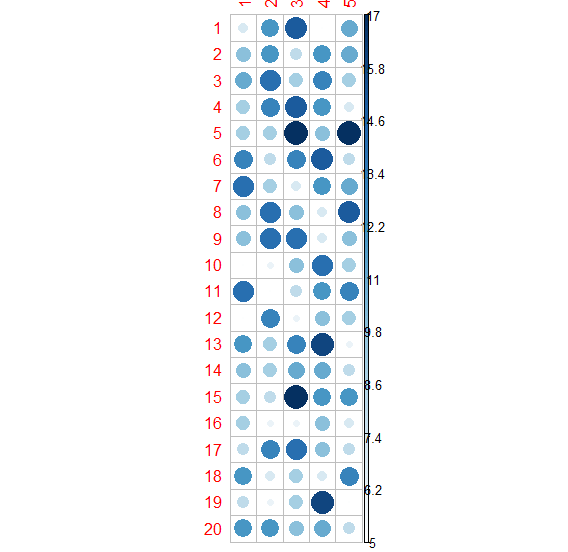# How to create matrix diagram in R?

R ProgrammingServer Side ProgrammingProgramming

To create matrix diagram, we can use corrplot function of corrplot function. For this purpose, we would need to set is.corr argument to FALSE so that the matrix values will be plotted in the diagram. Otherwise, the corrplot function requires correlation matrix instead of a matrix.

Check out the Example given below to understand how it works.

## Example

Following snippet creates a sample matrix −

M<-matrix(rpois(100,10),ncol=5)
M

The following matrix is created −

       [,1] [,2] [,3] [,4] [,5]
[1,]    7    12   15   5   11
[2,]   10    12    8  12   11
[3,]   11    14    9  13    9
[4,]    9    13   15  12    7
[5,]    9     9   17  10   17
[6,]   13     8   13  15    8
[7,]   14     9    7  12   11
[8,]   10    14   10   7   15
[9,]   10    14   14   7   10
[10,]   5     6   10  14    9
[11,]  14     5    8  12   13
[12,]   5    13    6  10    9
[13,]  12     9   13  16    6
[14,]  10     9   11  11    8
[15,]   9     8   17  12   12
[16,]   9     6    6  10    7
[17,]   8    13   14  10    8
[18,]  12     7    9   7   13
[19,]   8     6    9  16    5
[20,]  12    12   10  11    8

In order to load the corrplot package and to create a plot of matrix M on the above created data frame, add the following code to the above snippet −

M<-matrix(rpois(100,10),ncol=5)
library(corrplot)
corrplot(M,is.corr=FALSE)

## Output

If you execute all the above given snippets as a single program, it generates the following Output −